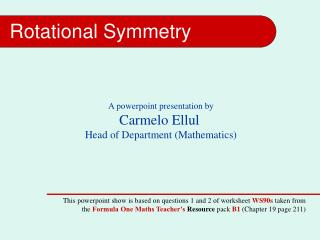DownloadDownload PresentationRotational Symmetry

# Rotational Symmetry

Télécharger la présentation## Rotational Symmetry

- - - - - - - - - - - - - - - - - - - - - - - - - - - E N D - - - - - - - - - - - - - - - - - - - - - - - - - - -
##### Presentation Transcript

1. Rotational Symmetry A powerpoint presentation by Carmelo Ellul Head of Department (Mathematics) This powerpoint show is based on questions 1 and 2 of worksheet WS90s taken from the Formula One Maths Teacher’s Resource pack B1 (Chapter 19 page 211)

2. Rotational Symmetry: WS90s Exercise 1: Which of these shapes have rotational symmetry? State the order of rotation for each shape. Rotational Symmetry of order 2 Rotational Symmetry of order 2 Rotational Symmetry of order 1 Rotational Symmetry of order 2 Rotational Symmetry of order 4

3. Rotational Symmetry: WS90s Exercise 2 (a): Complete the diagram so that the final shape has a rotational symmetry of order 2. 1 2 Order of Rotation

4. 2 Order of Rotation Rotational Symmetry: WS90s Exercise 2 (b), (c) Complete the diagrams so that the final shapes have a rotational symmetry of order 2.

5. 2 Order of Rotation Rotational Symmetry: WS90s Exercise 2 (d) Complete the diagram so that the final shape has a rotational symmetry of order 4. 4

6. 4 2 Order of Rotation Rotational Symmetry: WS90s Exercise 2 (e), (f): Complete the diagrams so that the final shapes have a rotational symmetry of order 4.

7. 2 2 Order of Rotation Order of Rotation Rotational Symmetry: WS90s Exercise 2 (e), (f) Complete the diagrams so that the final shapes have a rotational symmetry of order 8. 4 8 4 8 RESTART END SHOW